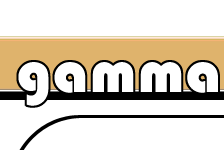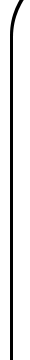# Geometric and Solid Modeling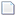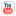The GAMMA research group is investigating techniques to perform efficient and accurate geometric computation. These include computation of Voronoi diagrams, medial axis, swept volumes, and complex shapes defined by Boolean operations.

### Model Synthesis

Paul Merrell and Dinesh Manocha

We present a method for procedurally modeling general complex 3D shapes. Our approach is targeted towards applications in digital entertainment and gaming and can automatically generate complex models of buildings, man-made structures, or urban datasets in a few minutes based on user-defined inputs.### Fast Distance Field and Voronoi Diagram Computation Using Graphics Hardware

Avneesh Sud, Naga K. Govindaraju, Kenneth E. Hoff III, Miguel A. Otaduy, Russell Gayle, Erik Andersen, Ilknur Kabul, Andrew Zaferakis, Ming C. Lin, and Dinesh Manocha

Given a set of geometric primitives (sites) and a distance function, the Voronoi diagram is a partition of space into cells such that point inside one cell are closer to one site. The distance field is a scalar field which gives the distance to the closest site at any point in space. Distance fields and Voronoi diagrams are closely related and one can be efficiently computed from the other. Graphics hardware can be used to efficiently compute a discrete distance field and approximate Voronoi diagrams. We present research on fast distance field and Voronoi diagram computation graphics hardware.

Project website...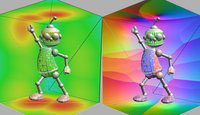### Fast 3D Distance Field Computation Using Graphics Hardware

Avneesh Sud, Miguel A. Otaduy, and Dinesh Manocha

We present an algorithm for fast computation of discretized 3D distance fields using graphics hardware. Given a set of primitives and a distance metric, our algorithm computes the distance field for each slice of a uniform spatial grid by rasterizing the distance functions of the primitives. We compute bounds on the spatial extent of the Voronoi region of each primitive and use them cull away many distance functions for each slice. Moreover, we exploit spatial coherence between adjacent slices and perform incremental computations to speed up the computation. The combination of culling and clamping techniques results in rendering relatively few distance functions for each slice and reduces the load on the graphics pipeline. Our algorithm is applicable to all geometric models and does not make any assumptions about connectivity or a manifold representation.

Project website...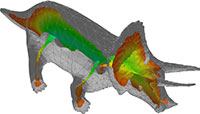### Fast Computation of Generalized Voronoi Diagrams Using Graphics Hardware

Kenneth E. Hoff III, Timothy Culver, John Keyser, Ming C. Lin, and Dinesh Manocha

We present a new approach for computing generalized Voronoi diagrams using interpolation-based polygon rasterization hardware. We compute a discrete Voronoi diagram by rendering a three dimensional distance mesh for each Voronoi site. The polygonal mesh is a bounded-error approximation of a (possibly) non-linear function of the distance between a site and a two-dimensional planar grid of sample points. For each sample point, we compute the closest site and the distance to that site using polygon scan-conversion and the Z-buffer depth comparison. We construct distance meshes for points, line segments, polygons, polyhedra, curves, and curved surfaces in two and three dimensions. We generalize to weighted and farthest-site Voronoi diagrams, and present efficient techniques for computing the Voronoi boundaries, Voronoi neighbors, and the Delaunay triangulation of points.

Project website...### Approximate Algorithms for Boolean Operations, Motion Planning, Sweeps, and Minkowski Sums

Gokul Varadhan, Shankar Krishnan, Young J. Kim, Sriram Thirthala Venkata, Liangjun Zhang, Ming C. Lin, and Dinesh Manocha

We are working on efficient algorithms for performing Boolean operations, motion planning, swept volume, and Minkowski sum computation. We employ distance field based volumetric techniques to perform these operations. The main advantage of these techniques is their efficiency. However, these techniques typically provide only an approximation to the exact surface without any guarantees on the quality of approximation. Compared to prior work, we focus on providing geometric and topological guarantees on our approximation. As a result, we are able to guarantee a good quality of approximation.

Project website...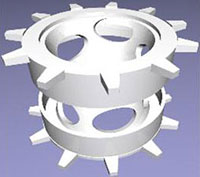### Fast Swept Volume Approximation of Complex Polyhedral Models

Young J. Kim, Gokul Varadhan, Ming C. Lin, and Dinesh Manocha

We present an efficient algorithm to approximate the swept volume (SV) of a complex polyhedron along a given trajectory. Given the boundary description of the polyhedron and a path specified as a parametric curve, our algorithm enumerates a superset of the boundary surfaces of SV. It consists of ruled and developable surface primitives, and the SV corresponds to the outer boundary of their arrangement. We approximate this boundary by using a five-stage pipeline. This includes computing a bounded-error approximation of each surface primitive, computing unsigned distance fields on a uniform grid, classifying all grid points using fast marching front propagation, isosurface reconstruction, and topological refinement.

Project website...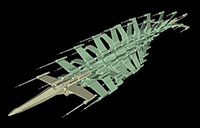### Homotopy-preserving Medial Axis Simplification

John Keyser, Timothy Culver, Shankar Krishnan, Mark Foskey, and Dinesh Manocha

We present an algorithm to compute a simplified medial axis of a polyhedron. Our simplification algorithm tends to remove unstable features of Blum's medial axis. Moreover, our algorithm preserves the topological structure of the original medial axis and ensures that the simplified medial axis has the same homotopy type as Blum's medial axis. We use the separation angle formed by connecting a point on the medial axis to closest points on the boundary as a measure of the stability of the medial axis at the point. The medial axis is decomposed into its parts that are the sheets, seams and junctions. We present a stability measure of each part of the medial axis based on separation angles and examine the relation between the stability measures of adjacent parts. Our simplification algorithm uses iterative pruning of the parts based on efficient local tests.

Project website...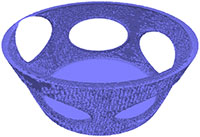### Efficient Computation of a Simplified Medial Axis

Mark Foskey, Ming C, Lin, and Dinesh Manocha

We construct an approximate simplified medial axis of a polyhedral model using a volumetric approach, accelerated by graphics hardware. For each voxel, the distance and direction to the nearest feature on the surface of a model is computed. If two neighboring voxels have direction vectors to their respective nearest neighbors that differ by a sufficiently large angle, we assume that the medial axis passes between the two voxels. A surface representation of the medial axis is then generated by a simple surface reconstruction technique.

Project website...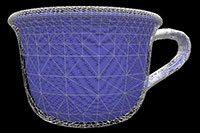### Accurate Computation of the Medial Axis of a Polyhedron

Timothy Culver, John Keyser, and Dinesh Manocha

We present an accurate algorithm to compute the internal Voronoi diagram and medial axis of a three-dimensional polyhedron. It uses exact arithmetic and exact representations for accurate computation of the medial axis. The algorithm works by recursively finding neighboring junctions along the seam curves. To speed up the computation, we have designed specialized algorithms for fast computation with algebraic curves and surfaces. These algorithms include lazy evaluation based on multivariate Sturm sequences, fast resultant computation, culling operations, and floating-point filters.

Project website...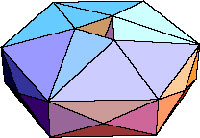### Exact Boundary Evaluation

John Keyser, Timothy Culver, Shankar Krishnan, Mark Foskey, and Dinesh Manocha

We present the ESOLID system that performs exact boundary evaluation of low-degree curved solids in reasonable amounts of time. ESOLID performs accurate Boolean operations using exact representations and exact computations throughout. The demands of exact computation require a different set of algorithms and efficiency improvements than those found in a traditional inexact floating point based modeler. We describe the system architecture, representations, and issues in implementing the algorithms.

Project website...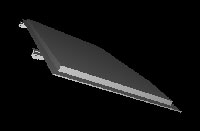### Past Members

• Erik Andersen
• Timothy Culver
• Mark Foskey
• Russell Gayle
• Naga K. Govindaraju
• Kenneth E. Hoff III
• Ilknur Kabul
• John Keyser
• Young J. Kim
• Shankar Krishnan
• Paul Merrell